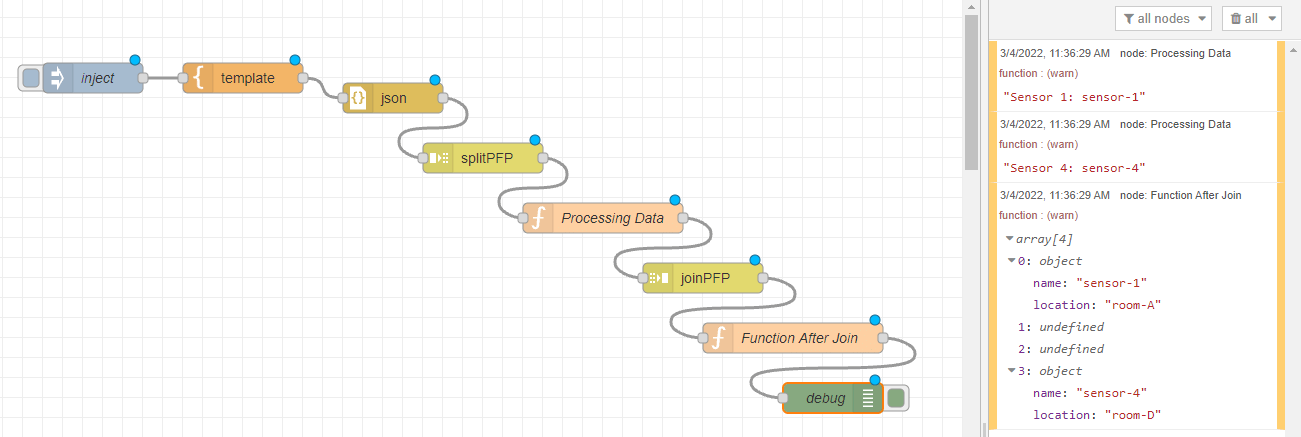# How to customize the Split node in Node-Red in a way not to send all msgs at once?

I am trying to customize the Split node in Node-Red in a way to send the next message only when the first arrives to the Join node; as I am doing some processing in between, and would like to process each msg separately before joining them.

So I have cloned the Split node from Node-Red project, and at the part where the splitting of an array happens; I register listeners to events (random IDs generated by the original Split node).

```else if (Array.isArray(msg.payload)) { // then split array into messages
msg.parts.type = 'array';
var count = msg.payload.length / node.arraySplt;
if (Math.floor(count) !== count) {
count = Math.ceil(count);
}
msg.parts.count = count;
var pos = 0;
msg.parts.len = node.arraySplt;
for (let i = 0; i < count; i++) {
msg.payload = data.slice(pos, pos + node.arraySplt);
if (node.arraySplt === 1) {
}
msg.parts.index = i;
pos += node.arraySplt;
if (i === 0) {
send(RED.util.cloneMessage(msg));
continue;
}
let eventName = msg.parts.id + '-' + i;
}
done();
}```

My handler function

```function addHandler(eventName, send, msg) {
send(RED.util.cloneMessage(msg));
});
}```

And at the Join node (which is in the same js file)

```// commented below is the original code of the join function
// if ((tcnt > 0 && group.currentCount > tcnt) || msg.hasOwnProperty('complete')) {
//     completeSend(partId);
//     return;
// } else
if (msg.parts.index <= (msg.parts.count - 2)) {
let eventName = msg.parts.id + '-' + (parseInt(msg.parts.index) + 1);
RED.events.emit(eventName);
} else if (msg.parts.index >= (msg.parts.count - 1)) {
completeSend(partId);
return;
}```

However, this would send the first msg (as I directly send it, and not through an event), and the last msg only; it would skip whatever in between.Contents

### Method 1

Modifying the split node is the wrong way to do this.

There are rate limiting nodes that will do this for you. e.g. https://flows.nodered.org/node/node-red-contrib-semaphore

Or you can use the delay node with it’s release function